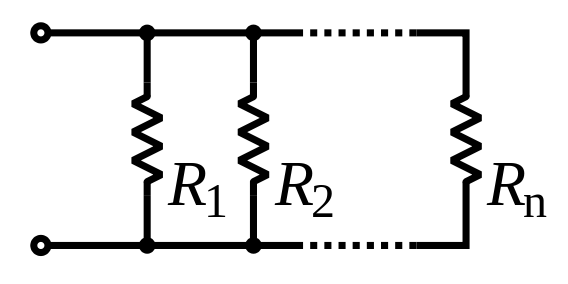# Problem 53770. Total resistance of resistors in parallel

What is the total resistance of a number of resistors in parallel?
A vector R contains the resistances (in Ohm) of n resistors, which are connected in parallel:Your function should take the vector R as its input and return the total resistance (in Ohm) of the parallel resistors.

### Solution Stats

59.3% Correct | 40.7% Incorrect
Last Solution submitted on Oct 17, 2023

### Community Treasure Hunt

Find the treasures in MATLAB Central and discover how the community can help you!

Start Hunting!